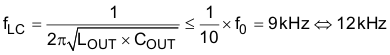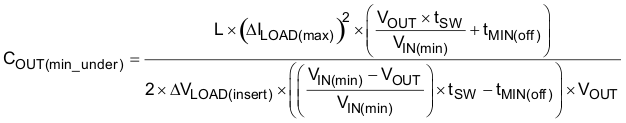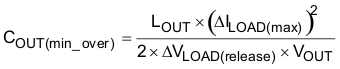SLUSDW9A June   2020  – June 2020

PRODUCTION DATA.

1. Features
2. Applications
3. Description
1.     Device Images
4. Revision History
5. Pin Configuration and Functions
6. Specifications
7. Detailed Description
8. Application and Implementation
9. Typical Applications
10. 10Power Supply Recommendations
11. 11Layout
12. 12Device and Documentation Support
13. 13Mechanical, Packaging, and Orderable Information
1. 13.1 Package Option Addendum

• RUK|20
• RUK|20

#### 9.2.7 Step Seven: Determine the Output Capacitance

The switching frequency for D-CAP2 mode is 600 kHz and it is generally recommend to have a unity gain crossover (f0) of 1/4 of the switching frequency, which is approximately 150kHz for the purpose of this application.

Given the range of the recommended unity gain frequency, the power stage design is flexible, as long as the LC double pole frequency is less than 10% of f0.

Equation 10.As long as the LC double pole frequency is designed to be less than 1/10 of f0, the internal compensation network provides sufficient phase boost at the unity gain crossover frequency in order for the converter to be stable with enough margin.

When the ESR frequency of the output bulk capacitor is in the vicinity of the unity gain crossover frequency of the loop, additional phase boost is achieved. This applies to POSCAP and/or SPCAP output capacitors.

When the ESR frequency of the output capacitor is beyond the unity gain crossover frequency of the loop, no additional phase boost is achieved. This applies to low/ultra low ESR output capacitors, such as MLCCs.

Equation 11 and Equation 12 can be used to estimate the amount of capacitance needed for a given dynamic load step/release. Note that there are other factors that may impact the amount of output capacitance for a specific design, such as ripple and stability. Equation 11 and Equation 12 are used only to estimate the transient requirement, the result should be used in conjuction with other factors of the design to determine the necessary output capacitance for the application.

Equation 11.Equation 12.Equation 11 and Equation 12 calculate the minimum COUT for meeting the transient requirement, which is 480 µF assuming ±7.5% voltage allowance for load step and release.